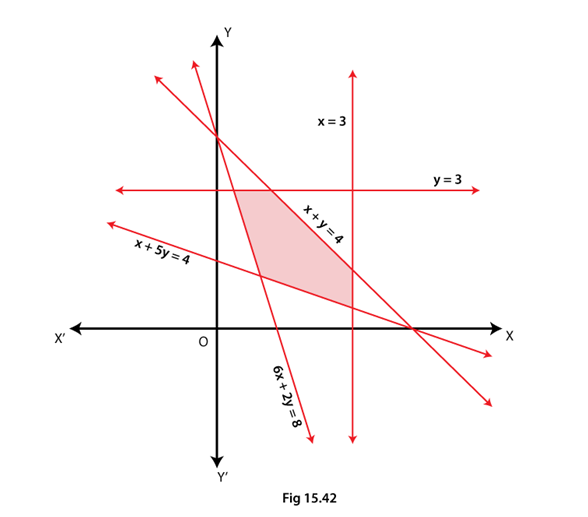• +91 9971497814
• info@interviewmaterial.com

# RD Chapter 15- Linear Inequations Ex-15.6 Interview Questions Answers

### Related Subjects

Question 1 :
Solve the following systems of linear inequations graphically.
(i) 2x + 3y ≤ 6, 3x + 2y ≤ 6, x ≥ 0, y ≥ 0
(ii) 2x + 3y ≤ 6, x + 4y ≤ 4, x ≥ 0, y ≥ 0
(iii) x – y ≤ 1, x + 2y ≤ 8, 2x + y ≥ 2, x ≥ 0, y ≥ 0
(iv) x + y ≥ 1, 7x + 9y ≤ 63, x ≤ 6, y ≤ 5, x ≥ 0, y ≥ 0
(v) 2x + 3y ≤ 35, y ≥ 3, x ≥ 2, x ≥ 0, y ≥ 0

(i) 2x + 3y ≤ 6, 3x + 2y ≤ 6, x ≥ 0, y ≥ 0
We shall plot the graph of the equation and shade the side containing solutions of the inequality,
You can choose any value but find the two mandatory values which are at x = 0 and y = 0, i.e., x and y–intercepts always,
2x + 3y ≤ 6
So when,
 x 0 1 3 y 2 1.33 0
3x + 2y ≤ 6
So when,
 x 0 1 2 y 3 1.5 0

x ≥ 0, y ≥ 0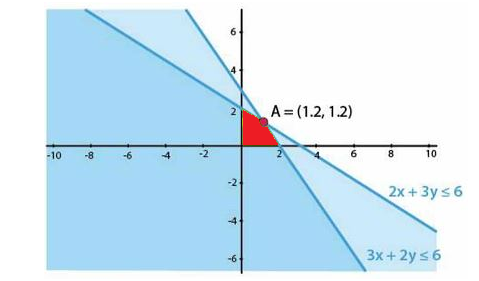(ii) 2x + 3y ≤ 6, x + 4y ≤ 4, x ≥ 0, y ≥ 0

We shall plot the graph of the equation and shade the side containing solutions of the inequality,

You can choose any value but find the two mandatory values which are at x = 0 and y = 0, i.e., x and y–intercepts always,

2x + 3y ≤ 6

So when,

 x 0 1 3 y 2 1.33 0

x + 4y ≤ 4

So when,

 x 0 2 4 y 1 0.5 0

x ≥ 0, y ≥ 0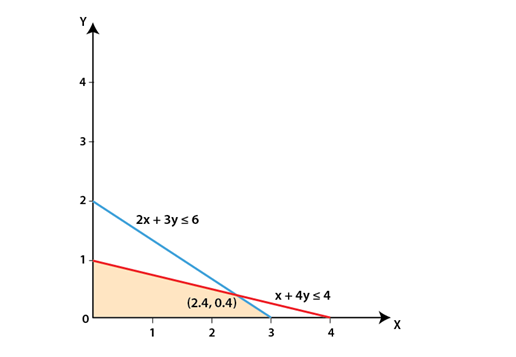(iii) x – y ≤ 1, x + 2y ≤ 8,2x + y ≥ 2, x ≥ 0, y ≥ 0

We shall plot thegraph of the equation and shade the side containing solutions of theinequality,

You can choose anyvalue but find the two mandatory values which are at x = 0 and y = 0, i.e., xand y–intercepts always,

x – y ≤ 1

So when,

 x 0 2 1 y -1 1 0

x + 2y≤ 8

So when,

 x 0 4 8 y 4 2 0

2x + y ≥ 2

So when,

 x 0 2 1 y 2 -2 0

x ≥ 0, y ≥ 0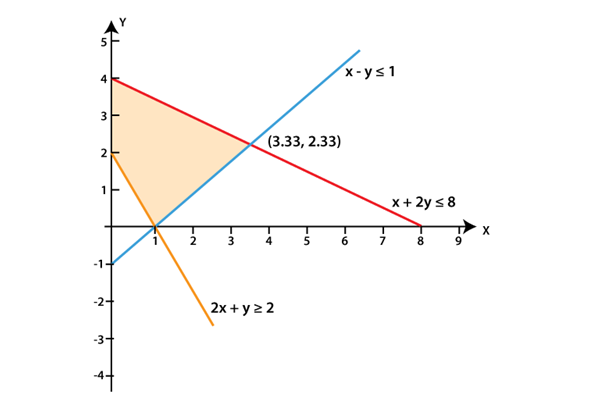(iv) x + y ≥ 1, 7x + 9y ≤63, x ≤ 6, y ≤ 5, x ≥ 0, y ≥ 0

We shall plot thegraph of the equation and shade the side containing solutions of theinequality,

You can choose anyvalue but find the two mandatory values which are at x = 0 and y = 0, i.e., xand y–intercepts always,

x + y ≥ 1

So when,

 x 0 2 1 y 1 -1 0

7x + 9y ≤ 63

So when,

 x 0 5 9 y 7 3.11 0

x ≤ 6, y ≤ 5 and x ≥0, y ≥ 0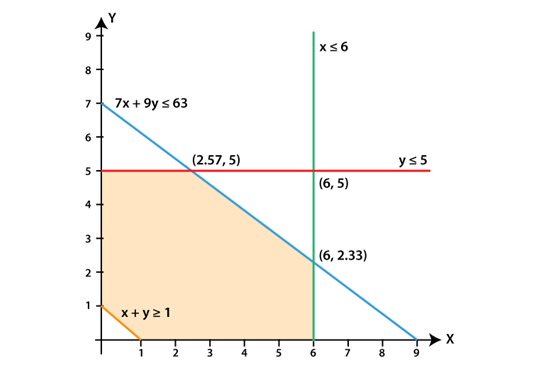(v) 2x + 3y ≤ 35, y ≥ 3, x≥ 2, x ≥ 0, y ≥ 0

We shall plot thegraph of the equation and shade the side containing solutions of theinequality,

You can choose anyvalue but find the two mandatory values which are at x = 0 and y = 0, i.e., xand y–intercepts always,

2x + 3y ≤ 35

So when,

 x 0 5 17.5 y 11.667 8.33 0

y ≥ 3, x ≥ 2, x ≥ 0, y≥ 0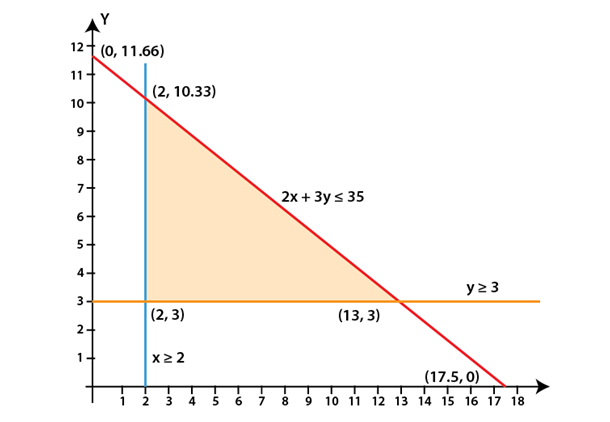Question 2 :
Show that the solution set of the following linear inequations is empty set:
(i) x – 2y ≥ 0, 2x – y ≤ –2, x ≥ 0, y ≥ 0
(ii) x + 2y ≤ 3, 3x + 4y ≥ 12, y ≥ 1, x ≥ 0, y ≥ 0

(i) x – 2y ≥ 0, 2x – y ≤–2, x ≥ 0, y ≥ 0

We shall plot thegraph of the equation and shade the side containing solutions of the inequality,

You can choose anyvalue but find the two mandatory values which are at x = 0 and y = 0, i.e., xand y–intercepts always,

x – 2y ≥ 0

So when,

 x 0 2 4 y 0 1 2

2x – y ≤ –2

So when,

 x 0 1 -1 y 2 4 0

x ≥ 0, y ≥ 0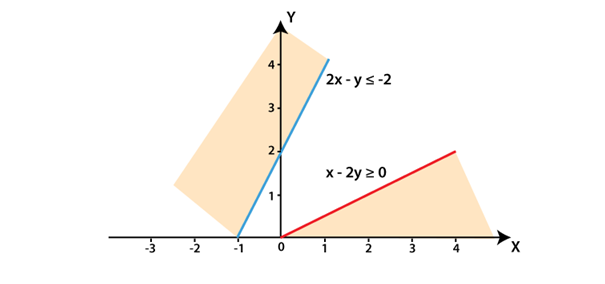The lines do notintersect each other for x ≥ 0, y ≥ 0

Hence, there is nosolution for the given inequations.

(ii) x + 2y ≤ 3, 3x +4y ≥ 12, y ≥ 1, x ≥ 0, y ≥ 0

We shall plot thegraph of the equation and shade the side containing solutions of theinequality,

You can choose anyvalue but find the two mandatory values which are at x = 0 and y = 0, i.e., xand y–intercepts always,

x + 2y ≤ 3

So when,

 x 0 1 3 y 1.5 1 0

3x + 4y ≥ 12

So when,

 x 0 2 4 y 3 1.5 0

y ≥ 1, x ≥ 0, y ≥ 0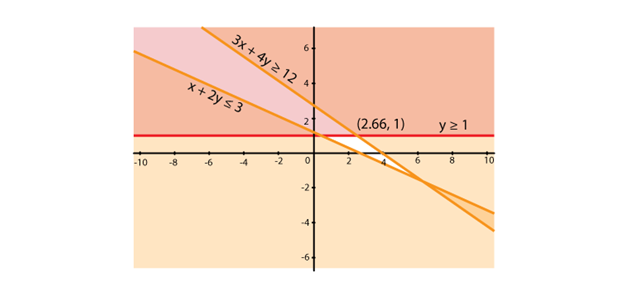Question 3 :

Find the linear inequations for which the shaded area in Fig. 15.41 isthe solution set. Draw the diagram of the solution set of the linearinequations.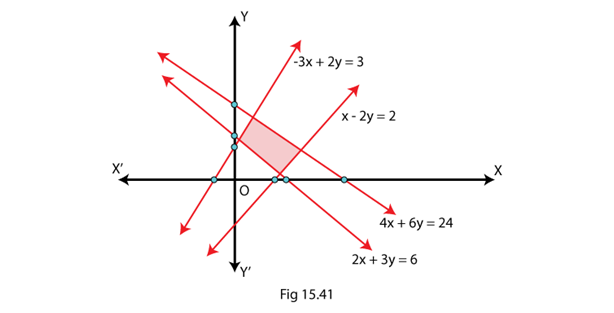Here, we shall applythe concept of a common solution area to find the signs of inequality by usingtheir given equations and the given common solution area (shaded part).

We know that,

If a line is in theform ax + by = c and c is positive constant. (In case of negative c, therule becomes opposite), so there are two cases which are,

If a line is above theorigin:

(i) If the shaded areais below the line then ax + by < c

(ii) If the shadedarea is above the line then ax + by > c

If a line is below theorigin then the rule becomes opposite.

So, according to therules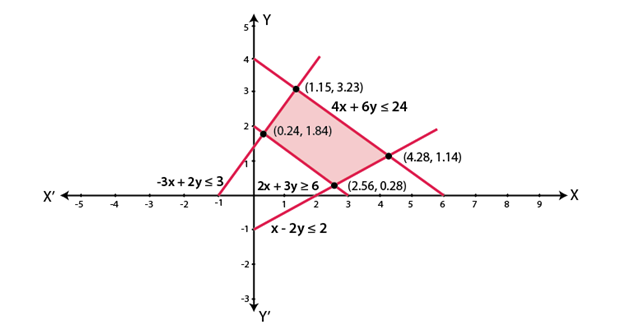Question 4 : Find the linear inequations for which the solution set is the shaded region given in Fig. 15.42.